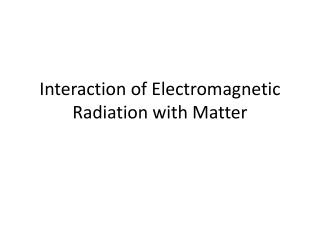DownloadDownload PresentationInteraction of Electromagnetic Radiation with Matter

# Interaction of Electromagnetic Radiation with Matter

Download Presentation## Interaction of Electromagnetic Radiation with Matter

- - - - - - - - - - - - - - - - - - - - - - - - - - - E N D - - - - - - - - - - - - - - - - - - - - - - - - - - -
##### Presentation Transcript

1. Interaction of Electromagnetic Radiation with Matter

2. Dielectric Constant and Atomic Polarizability • Under the action of the electric field, the positive and negative charges inside each atom are displaced from their equilibrium positions. • The induced dipole moment is p = αE Where α is atomic polarizability.

3. Dielectric Constant and Atomic Polarizability • The dielectric constant of a medium will depend on the manner in which the atoms are assembled. Let N be the number of atoms per unit volume. The polarization can be written approximately as P=Np=NαE=ε0ΧE Where Χ is susceptibility.

4. Dielectric Constant and Atomic Polarizability • The dielectric constant ε of the medium is • If the medium is nonmagnetic (i.e., μ=μ0), the index of refraction is given by

5. Classical Electron Model • In classical physics, we assume that the electrons are fastened elastically to the atoms and obey the following equation of motion • The electric field of the optical wave in the atom can be written as

6. Classical Electron Model • The equation has the following steady-state solution • The induced dipole moment is • The atomic polarizability is

7. Classical Electron Model • If there are N atoms per unit volume, the index of refraction of such a medium is • If the second term in this equation is small compared to 1, the index of refraction can be written as

8. Dispersion and Complex Refractive Index • From the equation • We can get that the index of refraction is higher for the waves with larger ω. • The phenomenon that the refractive index depends on frequency is called the phenomenon of dispersion.

9. Dispersion and Complex Refractive Index • The imaginary term iϒω in the equation accounts for the damping of electron motion and gives rise to the phenomenon of optical absorption.

10. Dispersion and Complex Refractive Index • The complex refractive index can be written as

11. Dispersion and Complex Refractive Index • For a wave written as • In which • Using the complex refractive index, the wave number K becomes

12. Dispersion and Complex Refractive Index • The electric field of the wave becomes

13. Optical Pulse and Group Velocity • In the pulsed mode, the pump energy can be concentrated into extremely short time durations, thereby increasing the peak power. • The propagation of a pulse due to dispersion can be described by representing the pulse as a sum of many plane-wave solutions of Maxwell’s equations.

14. Optical Pulse and Group Velocity • The scalar amplitude ψ(z,t) can be thought of as one of the components of electromagnetic field vectors. If A(k) denotes the amplitude of the plane-wave component with wave number k, the pulse ψ(z,t) can be written as

15. Optical Pulse and Group Velocity • A laser pulse is usually characterized by its center frequency ω0 or wave number k0and the frequency spread Δω or spread in wave number Δk. In other words, A(k) is sharply peaked around k0. We expand ω(k) around the value k0 in terms of a Taylor series:

16. Optical Pulse and Group Velocity • The ψ(z,t) becomes • The integral in above equation is a function of the composite variable [z – (dω/dk)0t] only and is called the envelope function

17. Optical Pulse and Group Velocity • The amplitude of the pulse can be written as • This shows that, apart from an overall phase, the laser pulse travels along undistorted in shape

18. Optical Pulse and Group Velocity • The group velocity of the pulse is • The group velocity represents the transport of energy.